Tutorials For Excel everyday with EVBA.info part 7[Free PDF ebook] - EBOOK VBA EXCELEVBA.info ( Ebook VBA Excel ) Welcome EVBA.info - Nothing Is Unable ... About Excel Tricks, Learning VBA Programming, Dedicated Software, Free Courses, Living Skills ...

## EVBA’s Tutorials For Excel:“Did You Know…”

Did you know:
You can insert a new worksheet by pressing `Shift+F11`. Immediately thereafter, to continue inserting as many new worksheets as you want, press the `F4` key.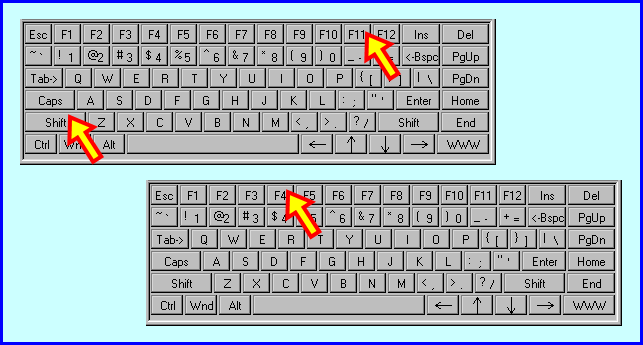Did you know:
You can enter any value in a cell, then select next cell below it, and reproduce that value by pressing `Ctrl+'` (Ctrl + apostrophe). Note that the value itself is reproduced but not the formatting.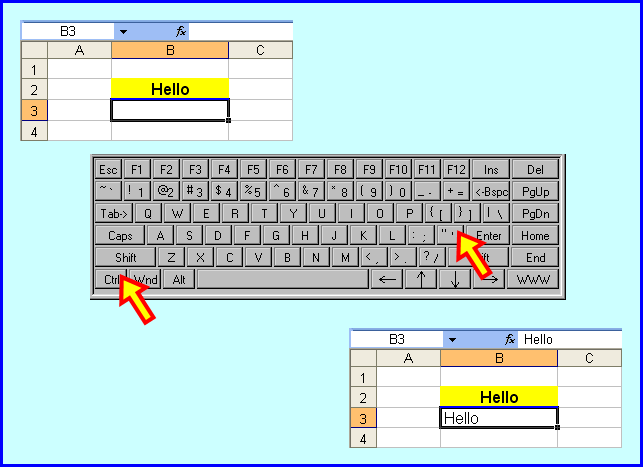Did you know:
Did you delete a sheet by mistake? You can restore it by immediately closing the workbook without saving it. When you reopen the workbook, your sheet will be restored.
Did you know:
• Copy – `Ctrl+C`
• Cut – `Ctrl+X`
• Paste with option to repeat – `Ctrl+V`
• Paste without option to repeat – `Enter`
Tom’s Tutorials For Excel: Linking a Drawing Object to a Cell
Here’s how you can link a cell’s value to be displayed inside a drawing object. In this example, a Text Box is being used, but this method will also work for Rectangles and other draw-able Forms shapes.
In Excel version 2003, find the Text Box icon on the Drawing toolbar by clicking View > Toolbars > Drawing to show that toolbar, then click onto the Text Box icon as shown.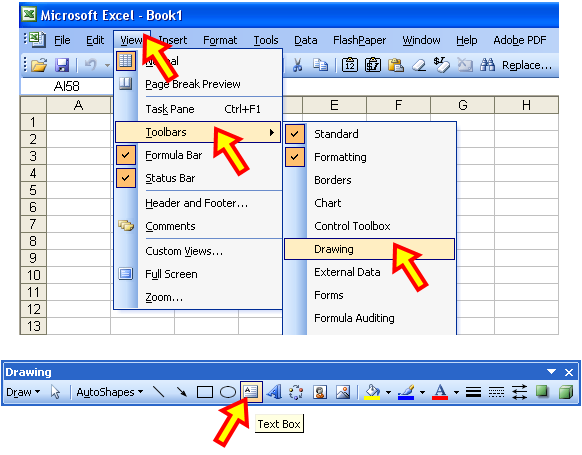As you see in the following pictures, draw the shape onto your worksheet, and select it. In the formula bar, enter a formula such as `=A1`, or whichever cell whose value you want the shape to show.
Whether the linked cell (A1 in this example) is meant for manual data entry, or it holds a formula, the shape will also show that cell’s contents.
Tom’s Tutorials For Excel: Validating Data Entry for xDivisible Number
Data Validation is an excellent way to control the entry of data in your worksheet to meet a certain condition. In this example, suppose you want to insure that numbers entered in the yellow cells are divisble by 5, as seen in the next picture. The following steps show how this can be done.
Step 3 of 5
In the Data Validation dialog box:
• Click onto the Settings tab.
• Click the down arrow for the Allow field.
• In the list of allowable categories, select Custom.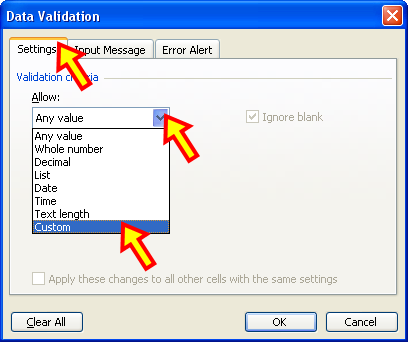Step 4 of 5
In the Formula field, enter the formula that is relative to your selected range.
In this example, that formula would be `=MOD(B3,5)=0`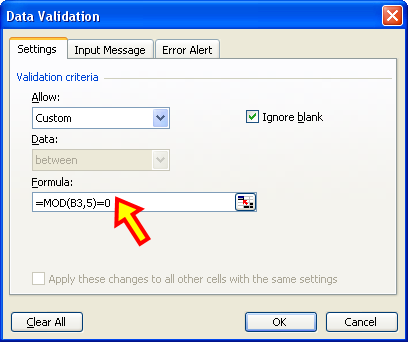Step 5 of 5
Still in the Data Validation dialog box:
• Click the Error Alert tab.
• Click to enter a checkmark in the box next to “Show error alert after invalid data is entered.”
• In the Style field, from the drop down list, select Stop.
• Enter the text for the Title of the error message you want to convey.
• Enter the text for the error message you want to convey.
• Click the OK button.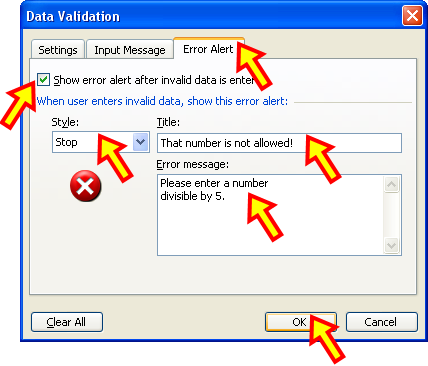Note, this example validates numbers divisible by 5, but you can adjust the Data Validation formula to allow other divisible-worthy numbers. For example, if you want to only allow entry of numbers divisible by 3, your Data Validation formula would be `=MOD(B3,3)=0`.
Tom’s Tutorials For Excel: Converting a trailing negative sign to a real negative number
As any Excel developer or workplace guru knows, raw downloads from external storage warehouses can throw some strange data at you. An example is a list of numbers, some of which are meant to be negative but are actually text values because of a trailing negative sign, as seen in the following Before and After pictures.
Here are the steps to convert those wannabe negative numbers to real negative numbers.
Step 1
Using a helper column, enter the conversion formula as shown in cell E3 copied down as needed:
`=IF(RIGHT(D3)="-",LEFT(D3,LEN(D3)-1)*-1,D3+0)`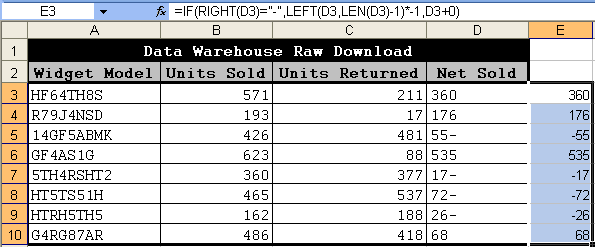Step 3
• Select the range of original numbers needing to be converted and paste special for values.
If you are using Excel version 2003:
From your keyboard press `Alt+E`, then `Enter`, then `S V`, then `Enter`.
If you are using Excel version 2007 or later:
From your keyboard press `Alt+E+S+V+Enter`.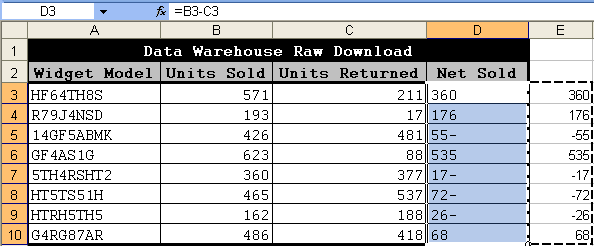Step 4
• Press the `ESC` key on your keyboard to exit Copy Mode.
• Not pictured but a good idea, delete the helper formulas, in this example E3:E10.
Tom’s Tutorials For Excel: Rounding Times by the Minute
Here are 3 formulas that round a time to its nearest minute, or up to the next minute, or down to the previous minute. This example uses the ten best times of the 2012 Boston Marathon. You’ll notice the number 1440 in the first formula, because 1440 minutes comprise a 24-hour day.
The first step is to enter your formula(s), starting with rounding the marathon times in column B to their nearest minute. That formula in cell C3 and copied down is
`=ROUND(\$B3*1440,0)/1440`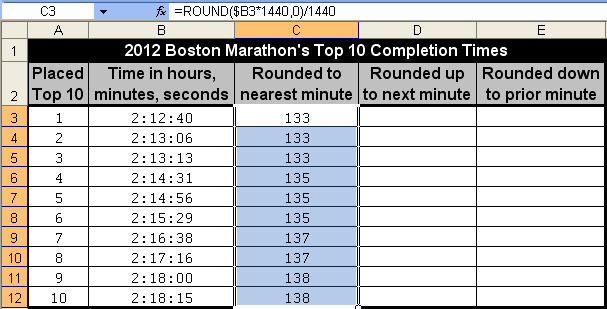The next formula rounds up the marathon times in column B to their next minute. That formula in cell D3 and copied down is
`=CEILING(\$B3,"00:01:00")`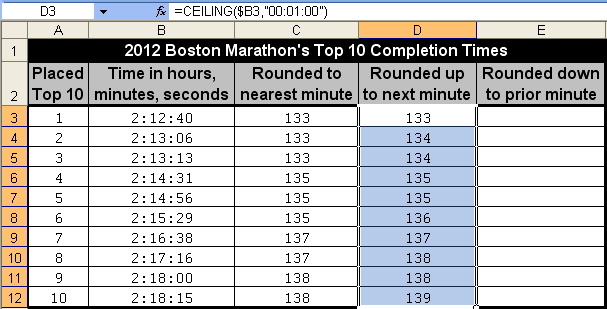The last formula rounds down the marathon times in column B to their lower previous minute. That formula in cell E3 and copied down is
`=FLOOR(\$B3,"00:01:00")`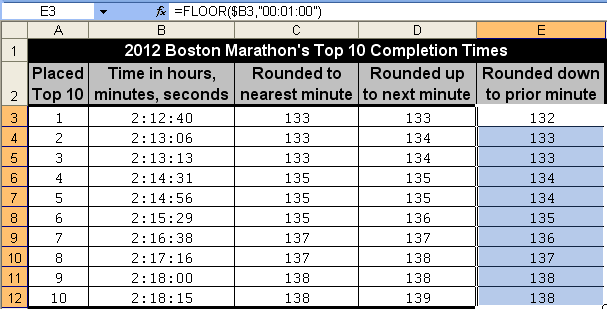After entering your rounding formulas, their returned values might look like a three-digit number. All that’s needed is to format those cells to make them look like the familiar, intuitive rounded results that you see in the first picture of this lesson.
In the Format Cells dialog box:
• Click onto the Number tab.
• In the Category pane, select Custom.
• In the Type field, enter the custom format `hh:mm:ss`
• Click the OK button.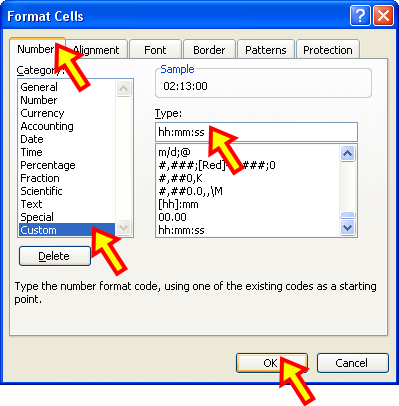Tom’s Tutorials For Excel: Rounding Elapsed Time by 5 Minutes
Here are 3 formulas that round an elapsed time by the 5-minute mark, in terms of nearest 5-minute mark, up to the next 5-minute mark, and down to the last (lower) 5-minute mark.
In the picture, notice the differences for each employee’s elapsed time in decimalized 5-minute segments, depending on if, and how, you decide to round or not round. The number 288 in the formulas relates to the count of 5-minute segements that comprise a full calendar day.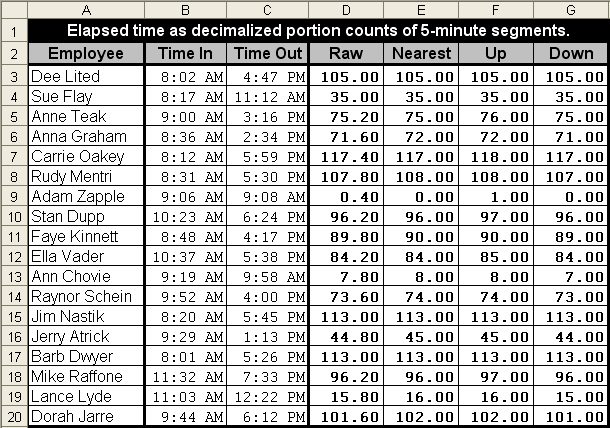The formula that subtracts the raw difference, entered in cell D3 and copied down:
`=(C3-B3)*288`
The subtraction formula rounding to the nearest 5-minute mark, entered in cell E3 and copied down:
`=ROUND((C3-B3)*288,0)`
The subtraction formula rounding up to the next 5-minute mark, entered in cell F3 and copied down:
`=CEILING((C3-B3)/(1/288),1)`
Tom’s Tutorials For Excel: Rounding Elapsed Time by the Quarter-Hour
Here are 3 formulas that round an elapsed time by the quarter-hour, in terms of nearest quarter-hour, up to the next quarter-hour, and down to the last (lower) quarter-hour.
In the picture, notice the differences for each employee’s elapsed time in decimalized quarter-hour segments, depending on if, and how, you decide to round or not round. The number 96 in the formulas relates to the count of quarter-hour (15-minute) segements in a full calendar day.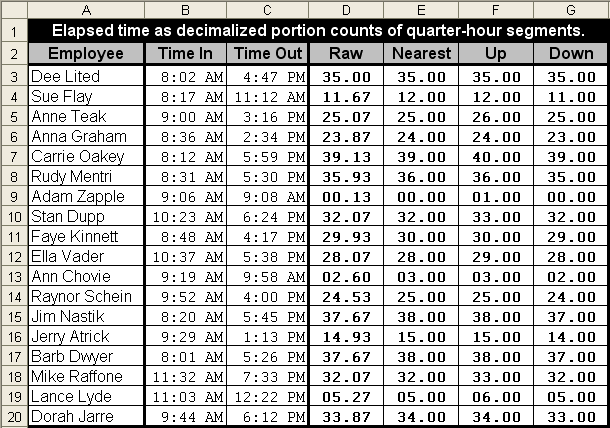The formula that subtracts the raw difference, entered in cell D3 and copied down:
`=(C3-B3)*96`
The subtraction formula rounding to the nearest quarter-hour, entered in cell E3 copied down:
`=ROUND((C3-B3)*96,0)`
The subtraction formula rounding up to the next quarter-hour, entered in cell F3 copied down:
`=CEILING((C3-B3)/(1/96),1)`
Tom’s Tutorials For Excel: Rounding Elapsed Time by the Half-Hour
Here are 3 formulas that round an elapsed time by the half-hour, in terms of nearest half-hour, up to the next half-hour, and down to the last (lower) half-hour.
In the picture, notice the differences for each employee’s elapsed time in decimalized half-hour segments, depending on if, and how, you decide to round or not round. The number 48 in the formulas relates to the count of half-hour (30-minute) segements that comprise a full calendar day.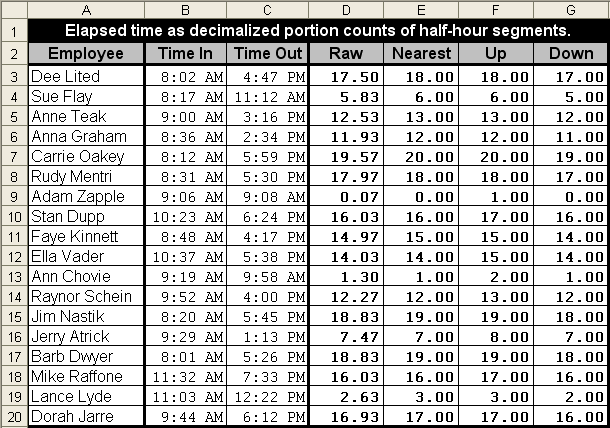The formula that subtracts the raw difference, entered in cell D3 and copied down:
`=(C3-B3)*48`
The subtraction formula that rounds to the nearest half-hour, entered in cell E3 and copied down:
`=ROUND((C3-B3)*48,0)`
The subtraction formula that rounds up to the next half-hour, entered in cell F3 and copied down:
`=CEILING((C3-B3)/(1/48),1)`
Tom’s Tutorials For Excel: Rounding Times of Day by 5 Minutes
Here are three pictures to show how a time of day can be rounded…
• To its nearest 5-minute mark.
• Up, to its next 5-minute mark.
• Down, to its last 5-minute mark.

Picture #1 – Rounding a time to its nearest 5-minute mark.
The formulas in range E3:F20 round their respective times in range B3:C20.
The formula in cell E3, which is copied across and down to cell F20 is
`=ROUND(B3*288,0)/288`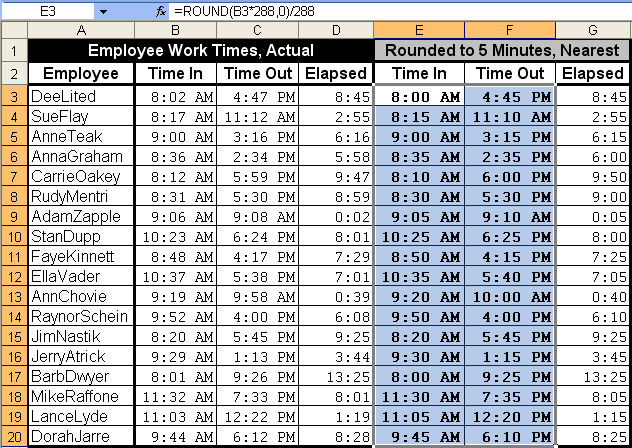Picture #2 – Rounding a time up to its next 5-minute mark.
The formulas in range E3:F20 round their respective times in range B3:C20.
The formula in cell E3, which is copied across and down to cell F20 is
`=CEILING(B3,0.5/144)`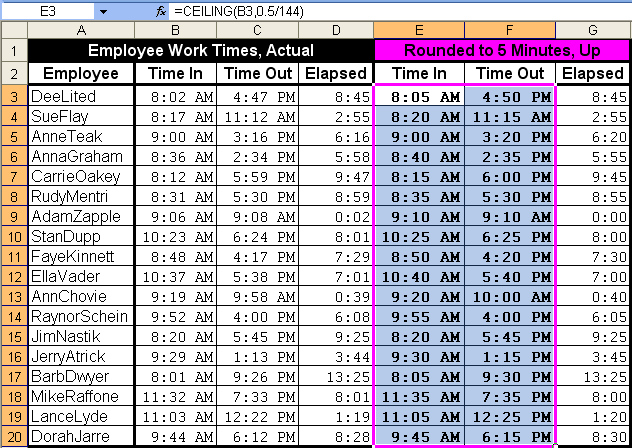Picture #3 – Rounding a time down to its last 5-minute mark.
The formulas in range E3:F20 round their respective times in range B3:C20.
The formula in cell E3, which is copied across and down to cell F20 is
`=FLOOR(B3,0.5/144)`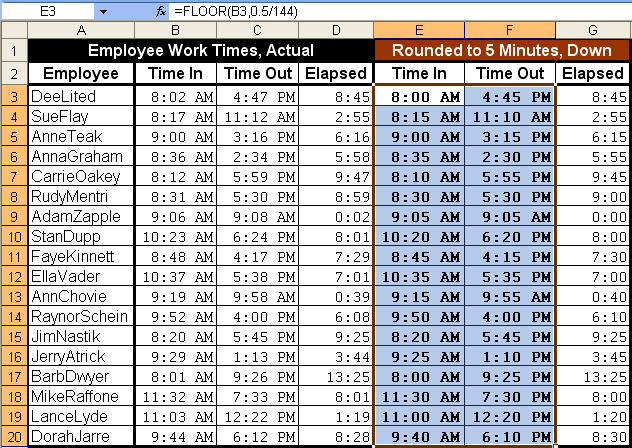Tom’s Tutorials For Excel: Rounding Times of Day by the Quarter Hour
Here are three pictures to show how a time of day can be rounded…
• To its nearest quarter-hour.
• Up, to its next quarter-hour.
• Down, to its last quarter-hour.

Picture #1 – Rounding a time to its nearest quarter-hour.
The formulas in range E3:F20 round their respective times in range B3:C20.
The formula in cell E3, which is copied across and down to cell F20 is
`=ROUND(B3*96,0)/96`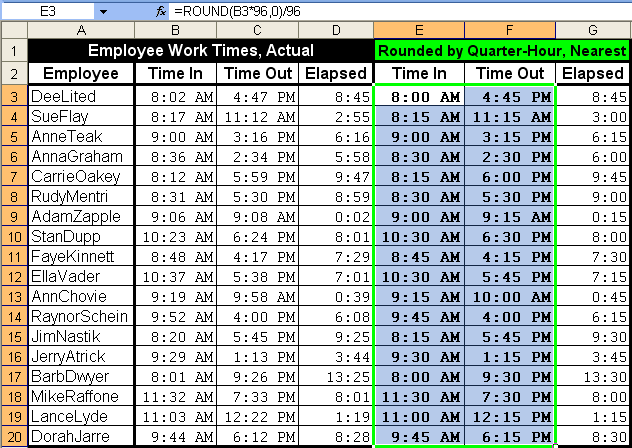Picture #2 – Rounding a time up to its next quarter-hour.
The formulas in range E3:F20 round their respective times in range B3:C20.
The formula in cell E3, which is copied across and down to cell F20 is
`=CEILING(B3,0.5/48)`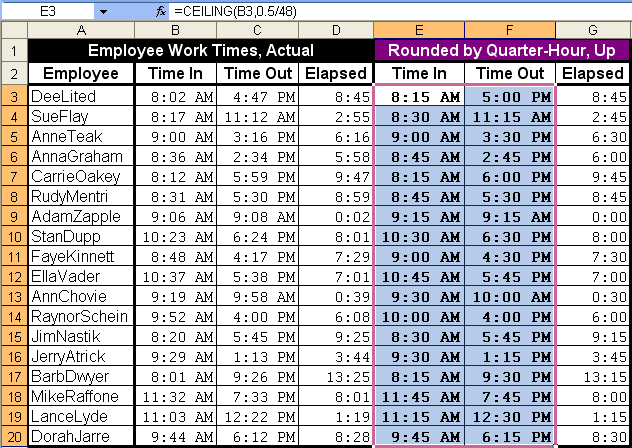Picture #3 – Rounding a time down to its last quarter-hour.
The formulas in range E3:F20 round their respective times in range B3:C20.
The formula in cell E3, which is copied across and down to cell F20 is
`=FLOOR(B3,0.5/48)`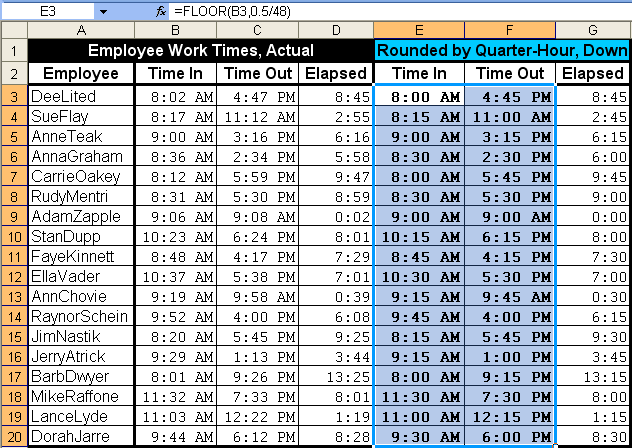📤You download App EVBA.info installed directly on the latest phone here : https://www.evba.info/p/app-evbainfo-setting-for-your-phone.html?m=1